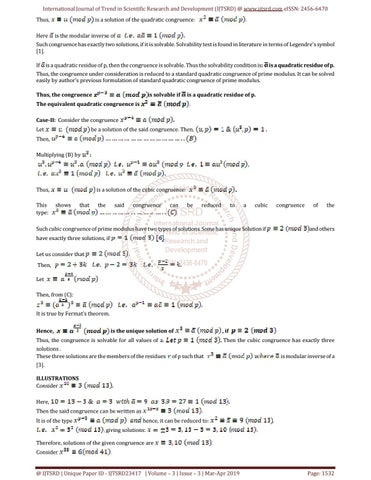International Journal of Trend in Scientific Research and Development (IJTSRD) @ www.ijtsrd.com eISSN: 2456-6470 Thus, Here

is a solution of the quadratic congruence: is the modular inverse of

Such congruence has exactly two solutions, if it is solvable. Solvability test is found in literature in terms of Legendre’s symbol . If

is a quadratic residue of p, then the congruence is solvable. Thus the solvability condition is:

is a quadratic residue of p.

Thus, the congruence under consideration is reduced to a standard quadratic congruence of prime modulus. It can be solved easily by author’s previous formulation of standard quadratic congruence of prime modulus. Thus, the congruence

is solvable if

is a quadratic residue of p.

The equivalent quadratic congruence is Case-II: Consider the congruence be a solution of the said congruence. Then,

Let Then, Multiplying (B) by

Thus, This type:

is a solution of the cubic congruence: shows

that

the

said

congruence

can

be

reduced

to

a

cubic

congruence

Such cubic congruence of prime modulus have two types of solutions. Some has unique Solution if have exactly three solutions, if

of

the

and others

.

Let us consider that Then, Let Then, from (C): It is true by Fermat’s theorem. Hence,

is the unique solution of

Thus, the congruence is solvable for all values of a. solutions . These three solutions are the members of the residues .

, if Then the cubic congruence has exactly three

of p such that

is modular inverse of a

ILLUSTRATIONS Consider Here, Then the said congruence can be written as It is of the type

hence, it can be reduced to:

.

giving solutions: Therefore, solutions of the given congruence are Consider

@ IJTSRD | Unique Paper ID - IJTSRD23417 | Volume – 3 | Issue – 3 | Mar-Apr 2019

Page: 1532

# RP 75 Formulation of two Special Classes of Standard Congruence of Prime Modulus of Higher Degree

by Prof. B M Roy "RP-75: Formulation of two Special Classes of Standard Congruence of Prime Modulus of Higher Degree" Published in Interna...

# RP 75 Formulation of two Special Classes of Standard Congruence of Prime Modulus of Higher Degree

by Prof. B M Roy "RP-75: Formulation of two Special Classes of Standard Congruence of Prime Modulus of Higher Degree" Published in Interna...